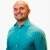# SAT Prep Determine number of intercepts of a polynomial factoredThis lesson will look at a SAT example question involving a factored polynomial. The given information will assist in determining the number of intercepts. This lesson will give a step by step explanation to better explain... This lesson will look at a SAT example question involving a factored polynomial. The given information will assist in determining the number of intercepts. This lesson will give a step by step explanation to better explain the concept.
More... Collapse
10 Views